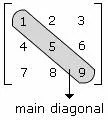By | 27.12.2016

# C++ Program to Find Sum of Main Diagonal Matrix

Write a C++ Program to Find Sum Above and Below of Main Diagonal Matrix. Here’s simple Program to Find Sum Above and Below of Main Diagonal Matrix in C Programming Language.

## Definition Of Main Diagonal : :

Main Diagonal of a matrix consists of the elements of a square from the upper left element proceeding to the down right element diagonally.

### More About Main Diagonal :

The sum of the elements of the main diagonal of a matrix is called Trace of the matrix.

### Examples of Main Diagonal :The elements 1, 5, and 9 are the elements of the main diagonal of a 3 × 3 matrix.

Below is the source code for C++ Program to Find Sum Above and Below of Main Diagonal Matrix which is successfully compiled and run on Windows System to produce desired output as shown below :

### SOURCE CODE : :

```/*   C++ Program to Find Sum Above and Below of Main Diagonal Matrix  */

#include<iostream>

using namespace std;

int main()
{
int arr,a=0,b=0,i,j,n;
cout<<"Enter size of matrix(max 5):";
cin>>n;
cout<<"Enter the matrix:\n";

for(i=0;i<n;++i)
for(j=0;j<n;++j)
cin>>arr[i][j];

for(i=0;i<n;++i)
for(j=0;j<n;++j)
if(j>i)
a+=arr[i][j];
else
if(i>j)
b+=arr[i][j];

cout<<"\nSum of elements above the diagonal:"<<a;
cout<<"\nSum of elements below the diagonal:"<<b;

return 0;
}```

### OUTPUT : :

```*************OUTPUT*****************

/* C++ Program to Find Sum Above and Below of Main Diagonal Matrix */

Enter size of matrix(max 5):3
Enter the matrix:
1
2
3
4
5
6
7
8
9

Sum of elements above the diagonal:11
Sum of elements below the diagonal:19```

If you found any error or any queries related to the above program or any questions or reviews , you wanna to ask from us ,you may Contact Us through our contact Page or you can also comment below in the comment section.We will try our best to reach upto you in the short interval.

5 1 vote
Article Rating
Category: Arrays Programs C++ Programming Tags: